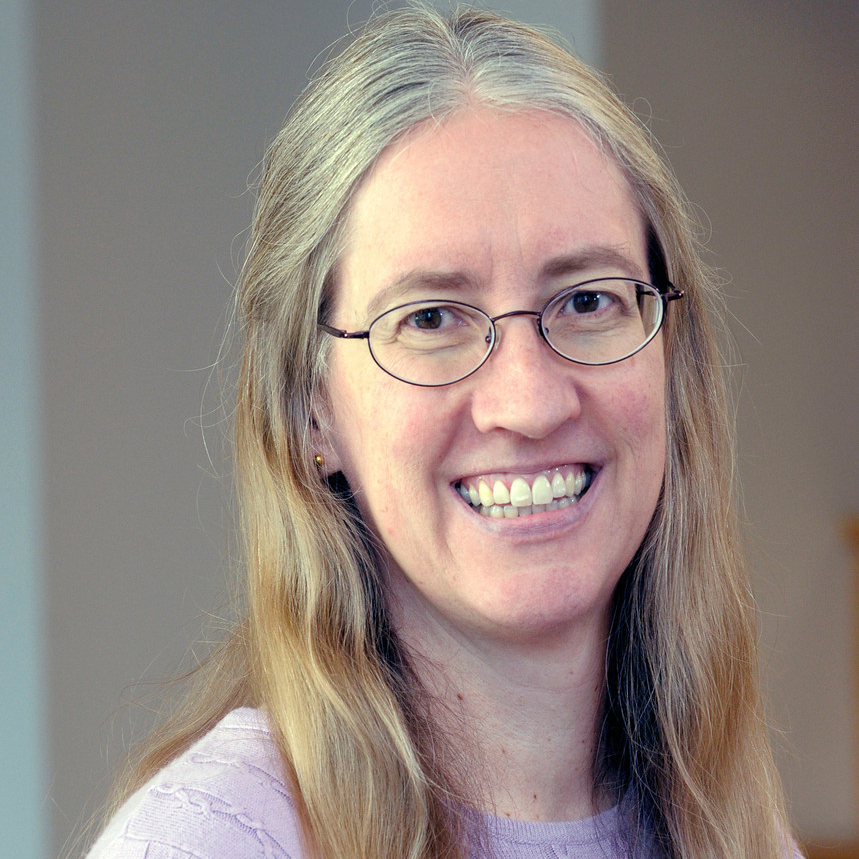# Helen G. Grundman

Professor Emeritus of Mathematics and Research Professor## Contact

Phone 5347
Website MathSciNet

## Education

Ph.D., UC Berkeley, 1989.

## Areas of Focus

Algebra, algebraic number theory, analytic number theory

## Biography

Video introduction to Professor Grundman

My mathematical research interests span a wide range of topics in the areas of algebra and number theory. My primary work is in the areas of algebraic number theory and computational algebraic geometry. Specifically, my research involves exploiting the connections between Hilbert modular varieties and certain algebraic number fields, studying the fields to determine classifications of the varieties. Additional research topics include:

• Galois embedding problems: characterizing fields that have field extensions with specified small 2-groups as Galois groups.
• Elementary number theory: discovering properties of the integers and interesting subsets of the integers, such as Niven numbers and happy numbers.
• Modular forms: studying properties of multiplier systems of functions similar to modular functions arising from considerations of modular equations.
• Diophantine equations: using algebraic number theory and computational methods to solve Diophantine equations over number rings.